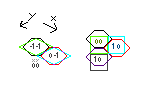# How do I implement Hexagonal Tilemaps

## Recommended Posts

Hi, I want to make my first Phaser game, a graphical Roguelike on a hexagonal grid.

I know, a square grid would be easier, but let me try it anyway.

How can I reuse as much code possible from the Phaser libraries (e.g. Tilemap) as possible?

I would like, for example, to use the functionality of these examples:

If I can use existing libraries, I would have less work, less bugs, and better performance. Performance isn't critical though and I might have less work if I implement everything myselft, rather than shoehorning the libraries to do something they weren't intended to.

• Collision detection isn't an issue (the player and the enemies are always standing in the middle of a cell).
• I would like to express North, North-East, South-East, South, South-West, and North-West as tuples of +1, -1 and 0.

Here is a doodle I made which explains how I could use isometric or rectangular grid "technology" (I hope it was worth the time to create it!).

(I wrote the x-coordinate first. I'm not sure if that's normal.)

Did anyone of you make a game with hex grid? How did you do it? What would you recommend to me? Is this the right forum? Do you have questions?##### Share on other sites

Thanks, but this isn't exactly what I was looking for. This is a tutorial about the math involved in hexagon.

I would like to know the most efficient way to render hexagonal tilemaps and how to reuse code from the libraries.

The tutorial uses a group of sprites with

`var hexagon = game.add.sprite(hexagonX,hexagonY,"hexagon");hexagonGroup.add(hexagon);`

Would everybody here recommend this approach? Then I would, for example, have to write my own loading and saving code.

Can I use a Tilemap somehow?

##### Share on other sites

I had this idea: I only use half of the "grid" for hexagon-tiles. Like this:

`-H-H-H-H-H-H-H-H-H-H-H-H-H-H-H-H-H-H`

Going North would mean (x+0, y-2), North-East (x+1, y-1), South-East (x+1, y-1), South (x+0, y+2), South-West (x-1, y+1), North-West (x-1, y-1).

I could store enemies in the empty tiles. Or I could declare them as above the other tiles.

One tile would be drawn with some "overhang", so it overlaps with the empty tiles, like this:

`.......................      .......===========............................      ....../...........\...........................      ...../.............\..........................      ..../...............\.....+------------+......      ...+------------+.../.....|............|......      ...|\...........|../......|............|......  ->  ...|.\..........|./.......|............|......      ...|..\.........|/........+------------+......      ...+---=========+......`

It's a hack but I'm very proud of it! When I'm done (which could take forever - you know how it goes) I'm going to post a link to the code here.

/edit: here it is: it works somewhat http://codepen.io/anon/pen/ogpKzO

##### Share on other sites

I love hex tiles! As for storing and rendering, it's VERY simple. Just use a plain old 2D array. The only trick is every other column (or row depending if you use pointy or flat hexes) is shifted over 50%.

Warning... code incoming!

/// NOTE: assumes flat-topped hexes, 60 pixels across and 52 pixels vertically
// draw the map
var WORLDX = 13;
var WORLDY = 11;
var TILESIZEX = 60;
var TILESIZEY = 52;

var offsetY = 0;    // odd columns are pushed down half a row
var spacingX = TILESIZEX * 0.75;
for (y = 0; y <= WORLDY; y++) {
for (x = 0; x <= WORLDX; x++) {
if ((x % 2) == 1) {
offsetY = TILESIZEY * 0.5;
} else {
offsetY = 0;
}

// load a sprite for this tile
var s = this.pGroup.create(x * spacingX, (y * TILESIZEY) + offsetY, "terrainTiles");
s.anchor.setTo(0.5, 0.5);
}
}

NOW... as far as north, south, etc. Two things are always constant. Up is always (x, y-1) and Down is always (x, y+1). The other directions switch depending on which column you're standing on.  You can do it like this:

/// ASSUMES I'm standing on tile x,y
/// where are my neighbors? Start up and go around clockwise.

if ((x % 2) == 1) {
// x is odd
numPulse += GemPulseInTile(x, y - 1);
numPulse += GemPulseInTile(x + 1, y);
numPulse += GemPulseInTile(x + 1, y + 1);
numPulse += GemPulseInTile(x, y + 1);
numPulse += GemPulseInTile(x - 1, y + 1);
numPulse += GemPulseInTile(x - 1, y);
} else {
// x is even
numPulse += GemPulseInTile(x, y - 1);
numPulse += GemPulseInTile(x + 1, y - 1);
numPulse += GemPulseInTile(x + 1, y);
numPulse += GemPulseInTile(x, y + 1);
numPulse += GemPulseInTile(x - 1, y);
numPulse += GemPulseInTile(x - 1, y - 1);
}

ps: that link Dario listed is exactly what you're looking for.